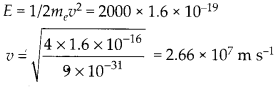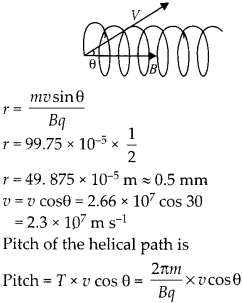Enlightened

# Question 19: NCERT Solutions for 12th Class Physics: Chapter 4-Moving Charges and Magnetism

• 0

Question 19: NCERT Solutions for 12th Class Physics: Chapter 4-Moving Charges and Magnetism

An electron emitted by a heated cathode and accelerated through a potential difference of 2.0 kV, enters a region with uniform magnetic field of 0.15 T. Determine the trajectory of the electron if the field (a) is transverse to its initial velocity, (b) makes an angle of 30° with the initial velocity.

Share

1. Solution:
A electron which is accelerated by a potential difference of 2.0 kV will have a kinetic energy gained 2000 eV.(a) When the electron enters in the uniform r magnetic field which is normal to the velocity of electron the electron follows a circular path r of radius.

(b) When the magnetic field makes an angle 30° with the initial velocity, the trajectory of the electron becomes helical. radius of the helical path isCheck the complete chapter with solutions.

NCERT Solutions for 12th Class Physics: Chapter 4-Moving Charges and Magnetism

• 0